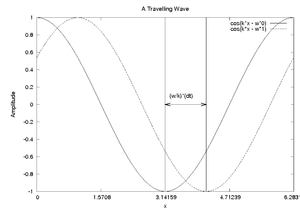# Waves and the Doppler Effect

## Travelling Waves

In phyics, we often encouter waves which, in addition to having an amplitude at each point in space, also appear to move in some direction over time. Such objects are called travelling waves. A simple sinusoidal travelling wave can be represented by the equation:

$y=cos(kx-\omega t)~$Where we call $k$the wavenumber, which has units of radians per metre ($m^{-1}$, and $\omega$is the angular frequency with units of radians per second $s^{-1}$. At any particular time, we can think of $-\omega t$as a phase shift $\phi ~$, and then this is the equation of a normal wave defined at every point along the x-axis:

$y=cos(kx+\phi )~$If we remember from our previous analysis of waves that $\phi ~$represents a shift of the waveform to the left or the right, it makes sense that when $\phi ~$depends on time, the wave with be continuously shifting to the left or the right.

Recall also that a positive $\phi ~$corresponds to the wave shifting to the left, and a negative $\phi ~$corresponds to the wave shifting to the right. Thinking about that, we can see why we use $cos(kx-\omega t)$and not $cos(kx+\omega t)$, because in this case whenever $k$and $\omega$are both positive, the wave moves to the right, which is the direction we usually associate with positive numbers.

### Direction of Travelling WavesTwo snapshots of a travelling wave at t=0 and t=1, with k=1 and w=1. Note that when the time changes by dt, the wave shifts to the right by the quantity w/k*dt.

It is pretty easy to tell by glancing at the eqution of a travelling wave which direction it is moving. To do this we need to recall the relationships between various numerical parameters of a wave. Recall that:

$k={\frac {2\pi }{\lambda }}$and

$\omega =2\pi f~$Where $\lambda$is the wavelength and $f$is the frequency in Hertz, or number of complete wavelengths per second. Since the wavelength is defined to be the length in metres of one wavelength, and the frequency is the number of complete wavelengths per second, it follows that the quantity $f\lambda$has units of metres per second and is the velocity of the wave. We can re-express $f$and $\lambda$in terms of the parameters in the equation by a travelling wave by using the eqations above:

$v=f\lambda ={\frac {\omega }{2\pi }}{\frac {2\pi }{k}}={\frac {\omega }{k}}$All this really means is that when you see the equation of a wave, say:

$cos(2x+3t)~$in order to determine which direction is is moving, it is sufficient to read off the values for $k$and $\omega$:

$k=2$$\omega =-3$thus:

$v={\frac {\omega }{k}}={\frac {-3}{2}}=-1.5m/s$The only possibly confusing part is to remember that there is a negative sign in front of the $\omega$in the general equation for the travelling wave, so you have to flip the sign of the number in front of $t$.

## Doppler Effect

The Doppler Effect is observed when you have sources of light, sound, or other kinds of waves being emitted from a source moving with respect to the observer. If you stand still and a source is emitting waves at a frequency $f$, you will observe the frequency $f$. If you or the source moves, then the frequency will shift based on your relative motion. The most common example of the doppler effect is a car with a siren speeding down the road as you stand on the sidewalk. The siren changes tone as it passed you from high to low (corresponding to the change in frequency of the sound waves from hight to low). The general equation for the observed frequency when a source and observer are moving is:

$f_{o}=f_{s}\left({\frac {v\pm v_{o}}{v\mp v_{s}}}\right)$$f_{o}~$is the frequency measured by the observer in Hertz ($Hz$).

$f_{s}~$is the emitted frequency (from the source) in Hertz ($Hz$).

$v~$is the speed of the wave (for sound, the speed of sound) in metres per second ($m/s$).

$v_{o}~$is the speed of the observer in meters per second ($m/s$).

$v_{s}~$is the speed of the source in meters per second ($m/s$).

The only confusing part of the doppler effect is choosing the right sign for $v_{o}$and $v_{s}$. There are is a simple convention which is described by two statements:

If the object and the observer are moving towards each other, $v_{o}$must be positive.


The sign for $v_{s}$is chosen to be consistent with the direction for $v_{o}$(i.e. if the observer is moving in the same direction as the source, then they will have the same sign. If they're travelling in opposite directions, they will have opposite sign). This sign convention is chosen because when the source and observer are moving towards each other, the observed frequency is higher than the emitted frequency. Similarly:

If the source and the observer are moving away from each other, $v_{o}$must be negative.


Again, the sign of $v_{s}$is chosen to be consistent with the sign of $v_{o}$. This convention ensures that when the observer and the source are moving away from each other the observed frequency is less than the emitted frequency.

Note, as alluded to above, that the source and the observer might be moving in the same direction, in which case you must determined their relative velocity to decide whether they will eventually meet or if they will drift apart. (In these cases $v_{o}$and $v_{s}$will have the same sign.

When doing problems based on waves and the Doppler effect, it is important to set out an expectation based on what you know. Typically the frequency of a wave will increase when either the observer or the source are moving towards each other and will decrease when either are moving away from each other. It should also be noted that these equations may be used multiple times when there are more than two source/observers (eg. sound waves from a source bouncing off a moving object prior to reaching a moving observer).